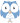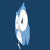On the next few pages, we're going to build up a query for dimensional analysis, piece by piece.

We're going to use f=ma as an example. For dimensional analysis, we need to work out, for each term in a formula, what its dimention is for the basic dimensions of QUDT, mass, length and time. Then we need to test whether the formula is balanced in each dimension; that is, the coefficient on the left side matches the coefficient on the right.

On this page, we break down complex quantities into their coefficients for each dimension in QUDT (this page does it for the Time dimension; the computation is the same for other dimensions). Then we come up with a way to indicate if a term is on the left or right hand side of a formula, and add up the balanced coefficient.

The queries in this section are based on the following data sets:

### Query ch14.8 Time dimension for each termThis query just works on the time dimension.

The quanties we are intersted in for f=ma are force, mass and acceleration. In QUDT, the quantity for force is qudt:DIM_SI-mkgs-2; the quantity for mass is qudt:DIM_SI-M; and the quantity for acceleration is qudt:DIM_SI-ms-2. In this query, we get the coefficient of each of these quantities in the Time dimension.### Query ch14.9 Balanced sum of Time exponentsIn this query, we make clever use of the VALUES keyword to specify, for each quantity, whether it is on the left or right side of a formula. ?term and ?side are bound together, to a quantity (like Mass) and a side of the question (left or right). Here we have mass and acceleration on the left, and force on the right (so we're really checking ma=f.

Once we have the time exponents for each quantity, we add up everything on the left and subtract everything on the right. A value of 0 means that the formula balances in this dimension. This is the case for Time in this formula.

Before moving on to Query 14-10, I suggest you update this query to work in the dimensions of Mass and then of Length, to see how it needs to change for different dimensions. Does it balance in every dimension?